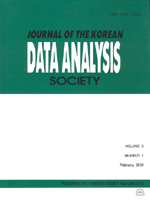상세검색
최근 검색어 전체 삭제
다국어입력
즐겨찾기0KCI등재 학술저널

# Optimality and Duality for Multiple Objective Nonconvex Optimization Problems

• 등재여부 : KCI등재
• 2007.06
• 1007 - 1016 (10 pages)

Optimality theorems of Fritz John type are established for multiple objective nonconvex optimization problems. Dual problems are given for these problems and it is shown that duality theorems hold without any form of constraint qualifications. We introduce some theorems about convex, pseudoconvex, and quasiconvex cases. Also, we explain of differences between these convex. The weak and strong duality relating (P) and (D) is proved in this paper. So, we recommend the differences among convex, pseudoconvex, and quasiconvex cases using the optimality and duality. Theorems of optimality and duality will give us information about convex.

1. Introduction and Preliminaries

2. Optimality Criteria

3. Duality Theorem

References